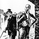1222 views
1222
MACD Zero lag Visual inchart view.

Threshold / Settings can be changed in Format view.

Threshold to be adapted depending on timeframe.
//@version=2
study('GreenMood inchart MACD Zero Lag', overlay=true)
// MACD VARIABLE
Range_Zerolag_BearMin = input(10)
Range_Zerolag_BearlMax = input(30)
Range_Zerolag_BullMin = input(-10)
Range_Zerolag_BulllMax = input(-30)
// MACD ZERO LAG  INPUT
source = close
fastLength = input(12, minval=1)
slowLength=input(26,minval=1)
signalLength=input(9,minval=1)
// MACD ZERO LAG  CALCULATION
// FAST LINE
ema1= ema(source, fastLength)
ema2 = ema(ema1,fastLength)
differenceFast = ema1 - ema2
zerolagEMA = ema1 + differenceFast
demaFast = (2 * ema1) - ema2
// SLOW LINE
emas1= ema(source , slowLength)
emas2 = ema(emas1 , slowLength)
differenceSlow = emas1 - emas2
zerolagslowMA = emas1 + differenceSlow
demaSlow = (2 * emas1) - emas2
//MACD LINE
ZeroLagMACD = demaFast - demaSlow
//SIGNAL LINE
emasig1 = ema(ZeroLagMACD, signalLength)
emasig2 = ema(emasig1, signalLength)
signal = (2 * emasig1) - emasig2
// MACD ZERO LAG  OUTPUT
plotredMACD = iff((crossover(signal, ZeroLagMACD) and ZeroLagMACD >Range_Zerolag_BearMin and ZeroLagMACD <Range_Zerolag_BearlMax), crossover(signal, ZeroLagMACD), na)
plotredSTRONG_MACD = iff((crossover(signal, ZeroLagMACD) and ZeroLagMACD >Range_Zerolag_BearlMax), crossover(signal, ZeroLagMACD), na)
plotgreenMACD = iff((crossover(ZeroLagMACD,signal) and ZeroLagMACD <=Range_Zerolag_BullMin and ZeroLagMACD >Range_Zerolag_BulllMax), crossover(ZeroLagMACD, signal), na)
plotgreenSTRONG_MACD = iff((crossover(ZeroLagMACD,signal) and ZeroLagMACD <Range_Zerolag_BulllMax), crossover(ZeroLagMACD, signal), na)

plotshape(plotredMACD, style=shape.labeldown, location=location.top, color=red, size=size.tiny, text="MACD Sell", textcolor=white)
plotshape(plotgreenMACD, style=shape.labelup, location=location.bottom, color=lime, size=size.tiny, text="MACD Buy", textcolor=white)
plotshape(plotgreenSTRONG_MACD, style=shape.labelup, location=location.belowbar, color=lime, size=size.tiny, text="MACD Strong Buy", textcolor=white)
plotshape(plotredSTRONG_MACD, style=shape.labeldown, location=location.abovebar, color=red, size=size.tiny, text="MACD Strong Sell", textcolor=white)Nice work, you think you could make strategy version of this? Would be neat to backtest.